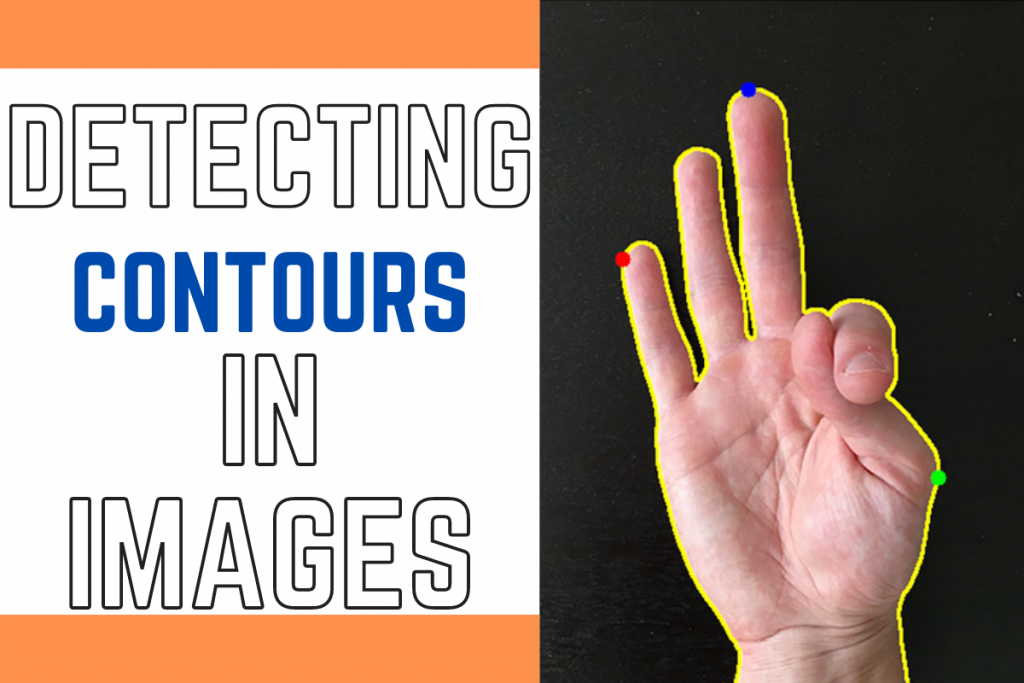# Python: Detecting ContoursHello fellow learner! Today we will learn about detecting contours in an image. Contours are defined as refined boundaries of objects and can be really helpful in detecting objects.

Recommended read: How to detect edges in Python?

## Detecting Contours using Python

So let’s get started with Detecting Contours for images using the OpenCV library in Python.

### 1. Importing Modules

First, we import the necessary modules which include OpenCV and matplotlib to plot the images on the screen.

```import cv2
import matplotlib.pyplot as plt
```

The next step includes loading the image from the file directory to our program using the `imread` function and then converting the image into `RGB` format.

We will plot the images in form of a `subplot` where the first image is the original loaded image. The code for the same is shown below.

```loaded_img = cv2.imread("image.jpg")

plt.figure(figsize=(20,20))

plt.subplot(2,2,1)
plt.title("Original Image")
plt.axis("off")
```

### 3. Converting image to GrayScale

For better detection of contours, we convert the image to a grayscale image by using `cvtColor` function. After converting the image to grayscale we plot it on the second subplot on the main plot.

```gray_image = cv2.cvtColor(loaded_img, cv2.COLOR_RGB2GRAY)
plt.subplot(2,2,2)
plt.title("Grayscale Image")
plt.imshow(gray_image,cmap="gray")
plt.axis("off")
```

### 4. Getting Binary Image

Next we convert the image into a binary image as it makes the image processing much much easier as it removes the unnecessary items from the image and focuses on the important objects only.

The code for the same is shown below. We will be plotting the binary image on the third slot on the main plot.

```_, binary_img = cv2.threshold(gray_image, 225, 255, cv2.THRESH_BINARY_INV)
plt.subplot(2,2,3)
plt.title("Binary Image")
plt.imshow(binary_img,cmap="gray")
plt.axis("off")
```

### 5. Detecting Contours

The final step is to detect contours using the `findContours` method of openCV library and then we draw the contours on the image.

We then plot all the images in the subplot, the code for the same is shown below.

```contours, hierarchy = cv2.findContours(binary_img, cv2.RETR_TREE, cv2.CHAIN_APPROX_SIMPLE)
final_image = cv2.drawContours(loaded_img, contours, -1, (0, 255, 0), 2)
plt.subplot(2,2,4)
plt.title("Contours detected Image")
plt.imshow(final_image,cmap="gray")
plt.axis("off")
```

## The Output Plot

The final output of the whole procedure explained above is shown below. You can see that the results are very accurate.

## The Final Code for Detecting Contours

```import cv2
import matplotlib.pyplot as plt

plt.figure(figsize=(20,20))

plt.subplot(2,2,1)
plt.title("Original Image")
plt.axis("off")

plt.subplot(2,2,2)
plt.title("Grayscale Image")
plt.imshow(gray_image,cmap="gray")
plt.axis("off")

_, binary_img = cv2.threshold(gray_image, 225, 255, cv2.THRESH_BINARY_INV)
plt.subplot(2,2,3)
plt.title("Binary Image")
plt.imshow(binary_img,cmap="gray")
plt.axis("off")

contours, hierarchy = cv2.findContours(binary_img, cv2.RETR_TREE, cv2.CHAIN_APPROX_SIMPLE)
final_image = cv2.drawContours(loaded_img, contours, -1, (0, 255, 0), 2)
plt.subplot(2,2,4)
plt.title("Contours detected Image")
plt.imshow(final_image,cmap="gray")
plt.axis("off")

plt.savefig('Contour_Detection_output_2.png', dpi = 1000,bbox_inches = 'tight')
plt.tight_layout()
plt.show()
```

I also tried the same code for a different image. The results are displayed below.